# Formulas

The SpreadsheetControl‘s built-in UI, the Formula Bar control, and the Formulas Ribbon tab commands that you can optionally add to your spreadsheet application allow end-users to easily manage formulas in their documents. For an example on how to create a formula bar and provide the Ribbon UI for the SpreadsheetControl, see the Getting Started topic.

## Formula Bar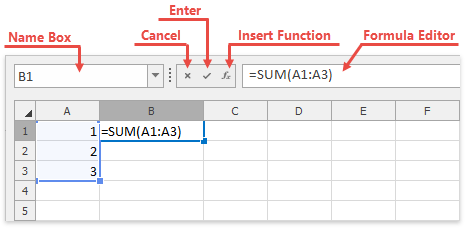The following SpreadsheetFormulaBar properties allow you to control the visibility of the formula bar’s elements:

Each button on the formula bar has a default action, as described in the table below. When an end-user clicks a particular button, a corresponding event is fired. Handle these events to override the default behavior or perform additional actions when buttons are clicked, if needed. To perform the action associated with a particular button in code, use the SpreadsheetFormulaBar.ExecuteResourceNavigatorAction method.

Button Description Event
Cancel Cancels the user input. SpreadsheetFormulaBar.CancelButtonClick
Enter Completes the cell entry and calculates the formula, if one has been entered. SpreadsheetFormulaBar.OkButtonClick
Insert Function Invokes the Insert Function or Function Arguments dialog to insert a function from the Function Library into a formula, or specify the function arguments. SpreadsheetFormulaBar.InsertFunctionButtonClick

## Enter Formulas

A formula is a string expression that starts with an equal sign (“=”). It can contain constants, operators, cell references, calls to functions, and names. An end-user can specify a cell formula in the cell’s in-place editor or the formula bar and use the following tools to enter different formula elements.

• Cell References

To enter cell references into formulas, end users can select necessary cell ranges directly in the current worksheet or another. They can use the mouse or keyboard to select cells. The SpreadsheetControl highlights the referenced cell ranges in different colors when users edit formulas.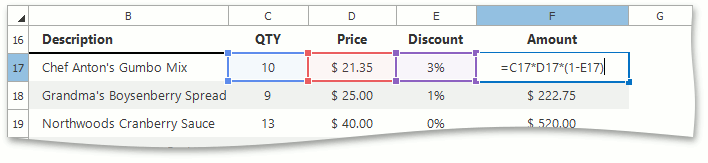• Defined Names

To insert a defined name into a formula, an end-user can type the name or select it from the Use in Formula list on the Formulas Ribbon tab in the Defined Names group.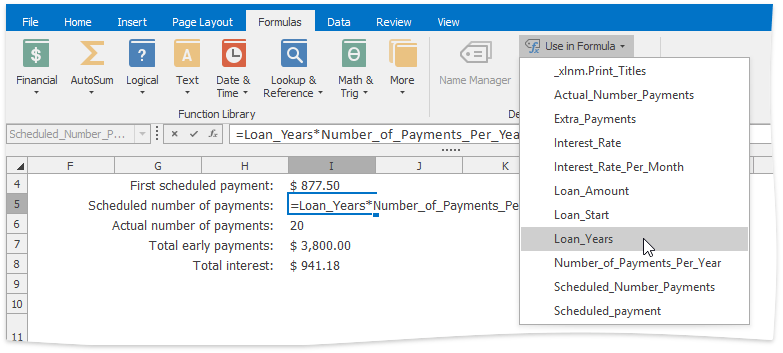• Functions

The SpreadsheetControl supports a variety of functions to be used in formulas. An end-user can insert a function in a formula in one of the following ways.

• Type the function name and enter its arguments in parentheses.
• Select the desired function from the Function Library group on the Formulas Ribbon tab. Frequently used functions such as Sum, Average, Count Numbers, Max and Min are also listed under the AutoSum button on the Home tab in the Editing group.
• Insert a function using the Insert Function dialog and specify the function parameters using the Function Arguments dialog.

The SpreadsheetControl also supports the Formula AutoComplete functionality, which displays a drop-down list of matching functions and names as you type a formula.

## Display Formulas in Cells

An end-user can specify whether cells should show formulas or calculated values. The Show Formulas button on the Formulas tab switches this behavior.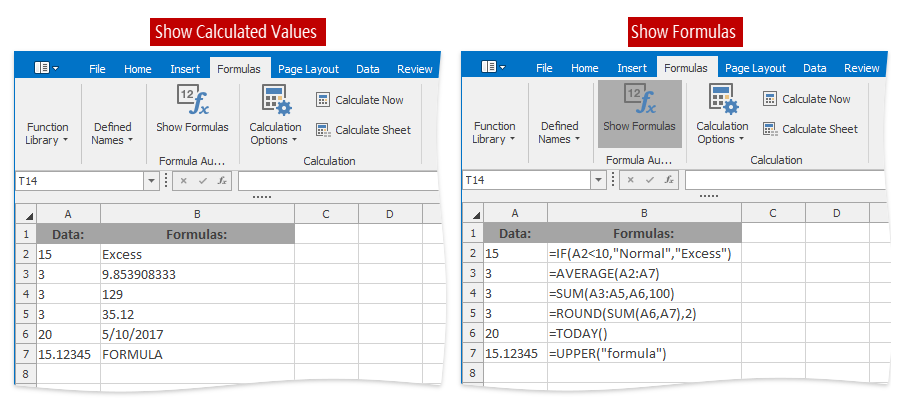## Options

The Calculation group on the Formulas tab contains buttons allowing an end-user to specify how formulas should be recalculated.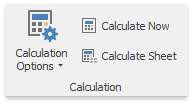There are two available modes of formula calculation (the Calculation Options sub-menu).

• Automatic - All dependent formulas are recalculated each time a cell value, formula, or name is changed. This is the default mode.
• Manual - Formulas are recalculated only when an end-user explicitly requests it.

When the Manual recalculation mode is activated, an end-user can manually recalculate formulas throughout the entire workbook (Calculate Now) or in the active worksheet only (Calculate Sheet).